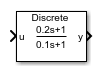•Libraries:
Simscape / Electrical / Control / General Control

## Description

The Lead-Lag (Discrete or Continuous) block implements a lead-lag compensator in conformance with IEEE 421.5-2016.

You can switch between continuous and discrete implementations of the block using the Sample time parameter.

### Equations

Continuous

To configure the compensator for continuous time, set the Sample time property to `0`. This representation is equivalent to the continuous transfer function:

`$G\left(s\right)=\frac{{T}_{1}s+1}{{T}_{2}s+1},$`

where:

• T1 is the lead time constant.

• T2 is the lag time constant.

From the preceeding transfer function, the compensator defining equations are:

`$\left\{\begin{array}{c}\stackrel{˙}{x}\left(t\right)=\frac{1}{{T}_{2}}\left(u\left(t\right)-x\left(t\right)\right)\\ y\left(t\right)=\frac{{T}_{1}}{{T}_{2}}u\left(t\right)+\left(1-\frac{{T}_{1}}{{T}_{2}}\right)x\left(t\right)\end{array}\text{\hspace{0.17em}}\text{\hspace{0.17em}}\text{\hspace{0.17em}}y\left(0\right)=x\left(0\right)={u}_{0},$`

where:

• u is the block input.

• x is the block state.

• y is the block output.

• t is the simulation time.

• u0 is the initial input to the block.

Discrete

To configure the compensator for discrete time, set the Sample time property to a positive, nonzero value, or to `-1` to inherit the sample time from an upstream block. The discrete representation is equivalent to the transfer function:

`$\frac{{T}_{1}z+\left({T}_{s}-{T}_{1}\right)}{{T}_{2}z+\left({T}_{s}-{T}_{2}\right)},$`

where:

• T1 is the lead time constant.

• T2 is the lag time constant.

• Ts is the compensator sample time.

From the discrete transfer function, the compensator equations are defined using the forward Euler method:

`$\left\{\begin{array}{c}x\left(n+1\right)=\left(1-\frac{{T}_{s}}{{T}_{2}}\right)x\left(n\right)+\left(\frac{{T}_{s}}{{T}_{2}}\right)u\left(n\right)\\ y\left(n\right)=\left(1-\frac{{T}_{1}}{{T}_{2}}\right)x\left(n\right)+\left(\frac{{T}_{1}}{{T}_{2}}\right)u\left(n\right)\end{array}\text{\hspace{0.17em}}\text{\hspace{0.17em}}\text{\hspace{0.17em}}y\left(0\right)=x\left(0\right)={u}_{0},$`

where:

• u is the block input.

• x is the state.

• y is the block output.

• n is the simulation time step.

• u0 is the initial input to the block.

### Initial Conditions

To specify the initial conditions of this block, set Initialization to:

• `Inherited from block input` — The block sets the state and output initial conditions to the initial input.

• `Specify as parameter` — The block sets the state initial condition to the value of Initial state.

### Limiting the Integral

Set the Upper saturation limit and Lower saturation limit parameters to use the anti-windup saturation method.

The anti-windup method limits the compensator state between the lower saturation limit A and upper saturation limit B:

`$A<=x<=B\text{\hspace{0.17em}}.$`

Because the state is limited, the output can respond immediately to a reversal of the input sign when the integral is saturated.

This block does not provide a windup saturation method. To use the windup saturation method, set the Upper saturation limit parameter to `inf`, the Lower saturation limit parameter to `-inf`, and attach a Saturation block to the output.

### Bypass Compensator Dynamics

Set the lag time constant to zero or to a value equal to that of the lead time constant to ignore the dynamics of the compensator. When bypassed, the block feeds the input directly to the output:

`$\begin{array}{c}{T}_{1}=0\\ {T}_{2}=0\\ {T}_{1}={T}_{2}\end{array}\right\}\text{\hspace{0.17em}}\text{\hspace{0.17em}}y=u\text{\hspace{0.17em}}.$`

In the continuous case, both the sample time and at least one time constant must be zero.

## Ports

### Input

expand all

Lead-lag compensator input signal. The block uses the input initial value to determine the state initial value.

Data Types: `single` | `double`

### Output

expand all

Data Types: `single` | `double`

## Parameters

expand all

Compensator lead time constant. To bypass the dynamics of the compensator. set this value to `0` or to the value of the Lag time constant, T2 parameter.

Compensator lag time constant. To bypass the dynamics of the compensator. set this value to `0` or to the value of the Lead time constant, T1 parameter.

Compensator upper state limit. Set this to `inf` for an unsaturated upper limit, or to a finite value to prevent upper windup of the system's integrator.

Compensator lower state limit. Set this to `-inf` for an unsaturated lower limit, or to a finite value to prevent lower windup of the system's integrator.

Specify the initial state condition for this block. For more information, see Initial Conditions.

Block initial state.

#### Dependencies

To enable this parameter, set Initialization to `Specify as parameter`.

Time between consecutive block executions. During execution, the block produces outputs and, if appropriate, updates its internal state. For more information, see What Is Sample Time? and Specify Sample Time.

For inherited discrete-time operation, set this parameter to `-1`. For discrete-time operation, set this parameter to a positive integer. For continuous-time operation, set this parameter to `0`.

If this block is in a masked subsystem or a variant subsystem that supports switching between continuous operation and discrete operation, promote this parameter to ensure correct switching between the continuous and discrete implementations of the block. For more information, see Promote Block Parameters on a Mask.

 IEEE Recommended Practice for Excitation System Models for Power System Stability Studies. IEEE Std 421.5-2016. Piscataway, NJ: IEEE-SA, 2016.